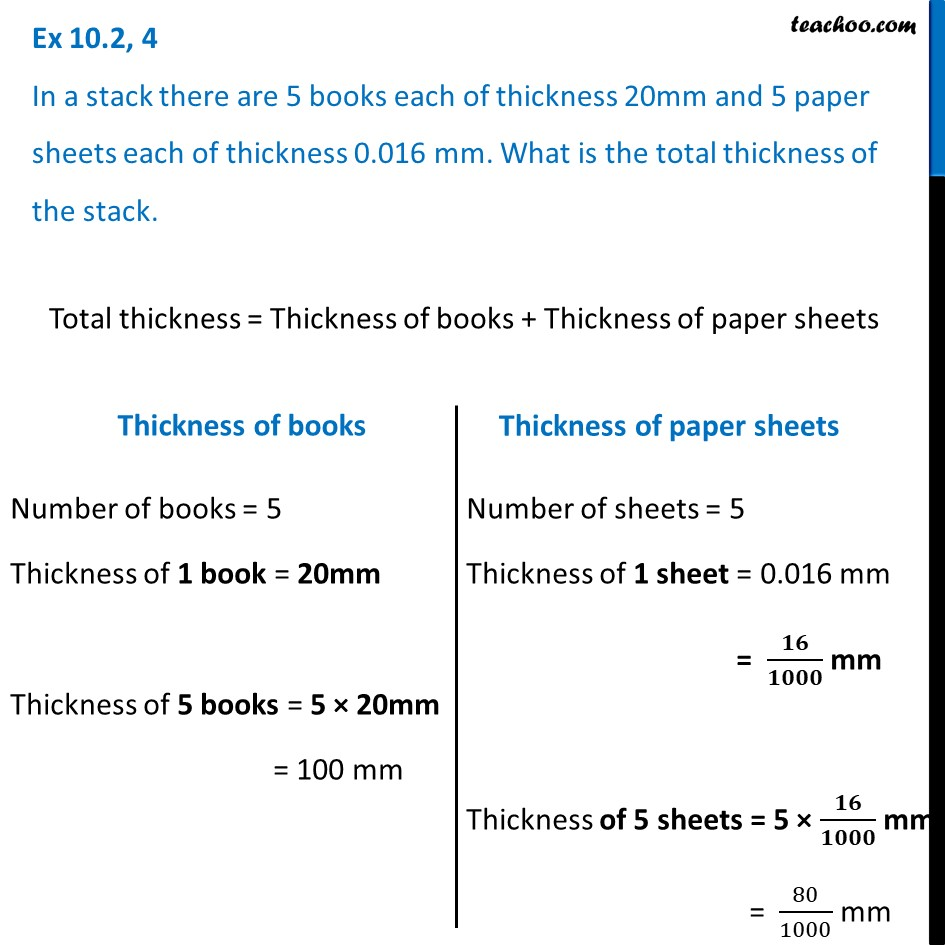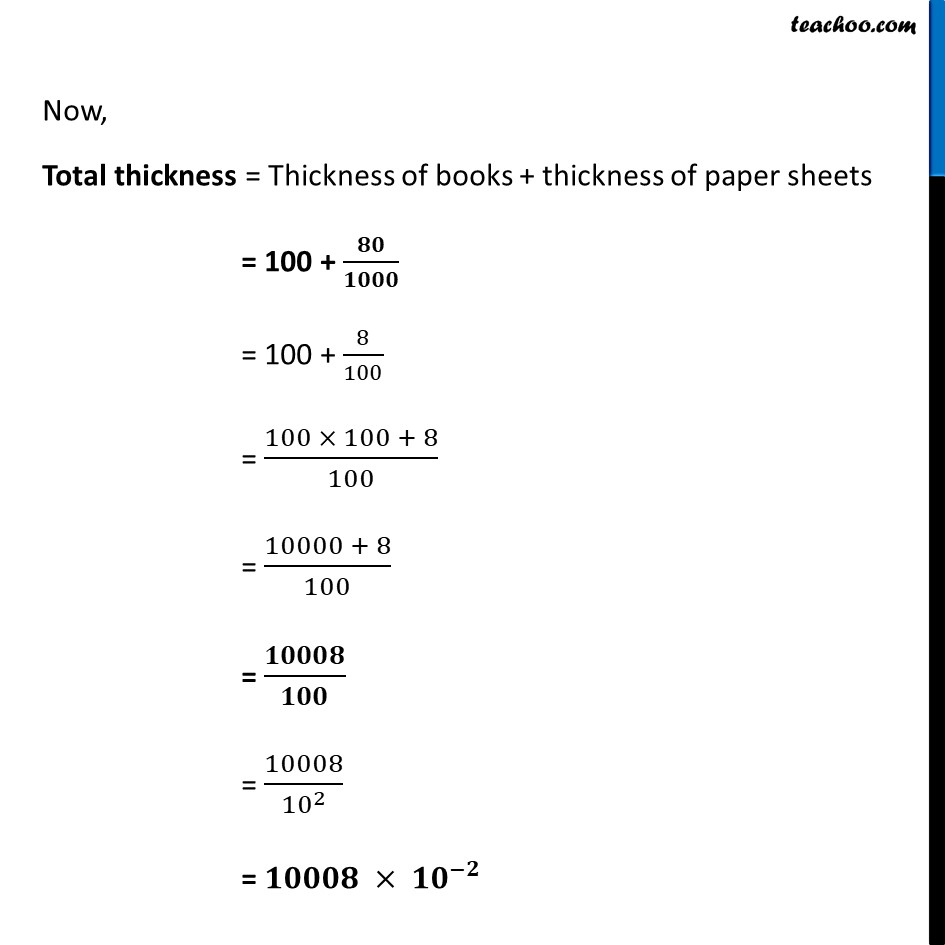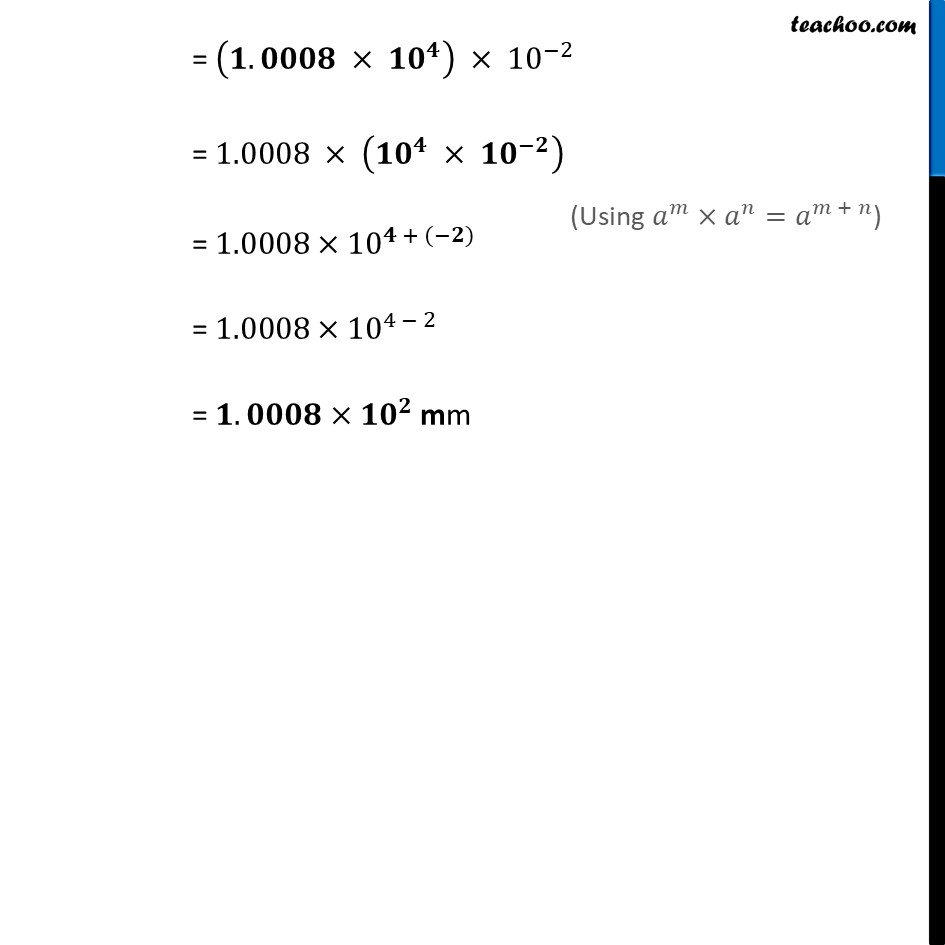Ex 10.2

Chapter 10 Class 8 Exponents and Powers
Serial order wiseLearn in your speed, with individual attention - Teachoo Maths 1-on-1 Class

### Transcript

Ex 10.2, 4 In a stack there are 5 books each of thickness 20mm and 5 paper sheets each of thickness 0.016 mm. What is the total thickness of the stack. Total thickness = Thickness of books + Thickness of paper sheets Number of books = 5 Thickness of 1 book = 20mm Thickness of 5 books = 5 × 20mm = 100 mm Number of sheets = 5 Thickness of 1 sheet = 0.016 mm = 𝟏𝟔/𝟏𝟎𝟎𝟎 mm Thickness of 5 sheets = 5 × 𝟏𝟔/𝟏𝟎𝟎𝟎 mm = 80/1000 mm